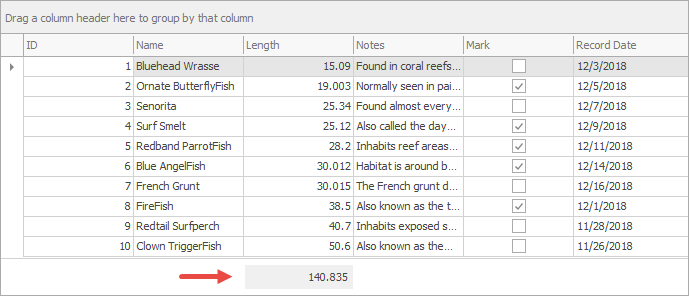# GridView.CustomSummaryCalculate Event

Allows you to manually calculate summaries. Fires for summaries whose SummaryItemType.Custom is SummaryItemType.Custom. Does not occur when the Data Grid functions in server mode.

Namespace: DevExpress.XtraGrid.Views.Grid

Assembly: DevExpress.XtraGrid.v19.1.dll

## Declaration

``````[DXCategory("Data")]
public event CustomSummaryEventHandler CustomSummaryCalculate``````

## Event Data

The CustomSummaryCalculate event's data class is CustomSummaryEventArgs. The following properties provide information specific to this event:

Property Description
FieldValue Gets the processed field value.
GroupLevel Gets the nested level of the group whose summary value is being calculated.
GroupRowHandle Gets a value identifying the group row whose child data rows are involved in summary calculation.
IsGroupSummary Gets whether a group summary value is being calculated.
IsTotalSummary Gets whether a total summary value is being calculated.
Item Gets a summary item whose value is being calculated.
Row Gets the currently processed row.
RowHandle Gets the handle of the processed row.
SummaryProcess Gets a value indicating calculation stage.
TotalValue Gets or sets the total summary value.
TotalValueReady Gets or sets whether the Calculation stage of the custom summary calculation process should be skipped.

The event data class exposes the following methods:

Method Description
GetGroupSummary(Int32, Object) Returns the value of the specified group summary for the specified group row.
GetValue(String) Returns the value in the specified field

## Remarks

If the GridSummaryItem.SummaryType property does not equal SummaryItemType.Custom, Data Grid automatically calculates summary values. Otherwise, the CustomSummaryCalculate event raises.

During the calculation process, the CustomSummaryCalculate event repeatedly fires multiple times.

Refer to the Working with Summaries in Code. Custom Summaries topic for additional information.

## Example

The example below creates a new custom summary items and populates it on the GridView.CustomSummaryCalculate event. You can test this sample in our DevExpress Demo Center (the link requires Demo Center of version 16.2 or newer to be installed).The custom summary item is located under the “Length” column. To add it, set up the GridColumn.SummaryItem property.

``````
gridView.OptionsView.ShowFooter = true;
GridColumn column = gridView.Columns["Length"];
column.SummaryItem.SummaryType = SummaryItemType.Custom;
``````

The CustomSummaryCalculate event handler checks the current calculation state by reading the CustomSummaryEventArgs.SummaryProcess value. Cell values are added to the total sum only if the corresponding cell under the “Mark” column is checked.

``````
double sum = 0;
gridView.CustomSummaryCalculate += (sender, e) => {
GridView view = sender as GridView;
if (e.IsTotalSummary && (e.Item as GridSummaryItem).FieldName=="Length") {
GridSummaryItem item = e.Item as GridSummaryItem;
if (item.FieldName == "Length") {
switch (e.SummaryProcess) {
case CustomSummaryProcess.Start:
sum = 0;
break;
case CustomSummaryProcess.Calculate:
bool shouldSum = (bool)view.GetRowCellValue(e.RowHandle, "Mark");
if (shouldSum) {
sum += (double)e.FieldValue;
}
break;
case CustomSummaryProcess.Finalize:
e.TotalValue = sum;
break;
}
}
}
};
``````

To automatically update the summary item when users check or clear the “Mark” column checkboxes, the GridView.UpdateTotalSummary method is called on the RepositoryItem.EditValueChanged event.

``````
RepositoryItemCheckEdit edit = gridView.Columns["Mark"].RealColumnEdit as RepositoryItemCheckEdit;
edit.EditValueChanged += (sender, e) => {
//Post an editor's value to a data source
gridView.PostEditor();
//Force calculation of the total summary
gridView.UpdateTotalSummary();
};
``````

The code below is a complete example code.

``````    // Handle this event to calculate summary values manually
double sum = 0;
gridView.CustomSummaryCalculate += (sender, e) => {
GridView view = sender as GridView;
if (e.IsTotalSummary && (e.Item as GridSummaryItem).FieldName=="Length") {
GridSummaryItem item = e.Item as GridSummaryItem;
if (item.FieldName == "Length") {
switch (e.SummaryProcess) {
case CustomSummaryProcess.Start:
sum = 0;
break;
case CustomSummaryProcess.Calculate:
bool shouldSum = (bool)view.GetRowCellValue(e.RowHandle, "Mark");
if (shouldSum) {
sum += (double)e.FieldValue;
}
break;
case CustomSummaryProcess.Finalize:
e.TotalValue = sum;
break;
}
}
}
};

gridView.OptionsView.ShowFooter = true;
GridColumn column = gridView.Columns["Length"];
column.SummaryItem.SummaryType = SummaryItemType.Custom;

RepositoryItemCheckEdit edit = gridView.Columns["Mark"].RealColumnEdit as RepositoryItemCheckEdit;
edit.EditValueChanged += (sender, e) => {
//Post an editor's value to a data source
gridView.PostEditor();
//Force calculation of the total summary
gridView.UpdateTotalSummary();
};
``````